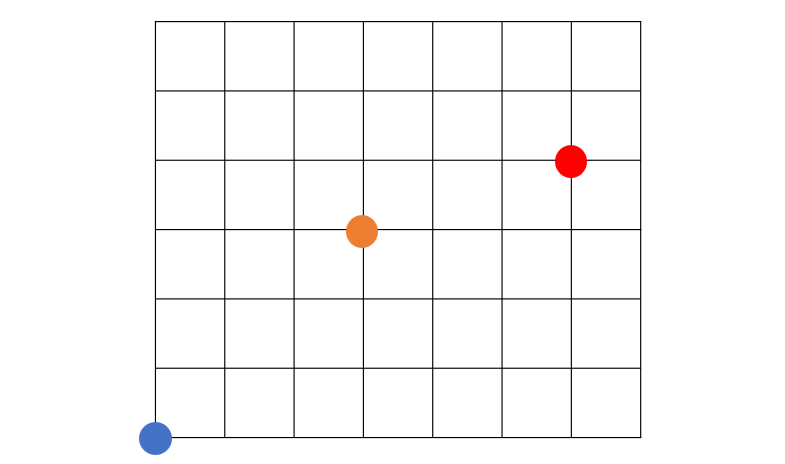By Long Luo

# Intuition

We can find that this is a graph theory problem with analysis.

Imagine the stone on the 2D coordinate plane as the vertex of the graph, If the x-coord or the y-coord of two stones are the same, there is an edge between them.

This can be show as follows:According to the rule that stones can be removed, we should remove those points that are in the same row or column with other points as late as possible. That is, the more points in the same row or column with point A, the later point A should be removed. In this way, we can delete as many points as possible through point A.

It can be found that all vertices in a connected graph can be deleted to only one vertex.Since these vertices are in a connected graph, all vertices of the connected graph can be traversed by DFS or BFS.

Therefore: the maximum number of stones that can be removed = the number of all stones - the number of connected components.

# DFS

## Analysis

• Time Complexity: O(n^2).
• Space Complexity: O(n).

# Union Find

## Analysis

• Time Complexity: $O(nlogn)$
• Space Complexity: $O(n)$

All suggestions are welcome.
If you have any query or suggestion please comment below.
Please upvote👍 if you like💗 it. Thank you:-)

Explore More Leetcode Solutions. 😉😃💗